# serial communication 8051 microcontroller using keil

In this tutorial, we will see a serial communication programming of 8051 microcontroller. when electronic devices communicate with each other, they can transfer data in two different ways. One is serial and the other one is parallel, When digital data is transferred serially, it is transmitted bit by bit, whereas in parallel transfer, many bits are transmitted at the same time. Though the parallel transfer of data is much faster but requires many wires. while a serial transfer is slower as compared to parallel transfer but requires few wires. Serial communication may be synchronous or asynchronous. In synchronous communication, the transmitter also transmits a clock along with data. This clock is used for synchronization between transmitter and receiver devices In an asynchronous transfer of data, there is no clock.

## Introduction to SERIAL COMMUNICATION with 8051 microcontroller

serial communication may be simplex, half-duplex or full-duplex. simplex communication means that data will be transmitted only in one direction while half-duplex means data will be transmitted in both directions but at one time, only one device can transmit, whereas full-duplex means data may be transmitted in both directions at one time, while one device is transmitting, it can also receive data transmitted from other devices at same time. As I have mentioned before, the transmitter and receiver are configured to communicate at some data transfer rate before communication starts. This data transfer rate or a number of bits transmitted per second is called the baud rate for handling serial communication.

Parallel communication is fast but it is not applicable for long distances (for printers). Moreover, it is also expensive. Serial is not much fast as parallel communication but it can deal with transmission of data over longer distances (for telephone line, ADC, DAC). It is also cheaper and requires less physical wires, that’s why we use serial communication. This article also deals with how to serially communicate in 8051 microcontroller.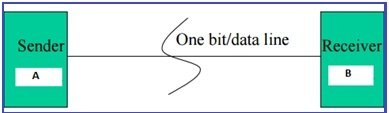## METHODS OF SERIAL COMMUNICATION

There are two methods of Serial Communication: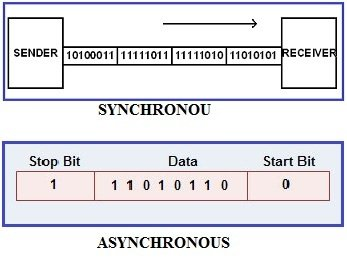SYNCHRONOUS: Transfer the block of data (characters) between sender and receiver spaced by fixed time interval.  This transmission is synchronized by an external clock.

ASYNCHRONOUS:  There is no clock involved here and transmission is synchronized by special signals along the transmission medium. It transfers a single byte at a time between sender and receiver along with inserting a start bit before each data character and a stop bit at its termination so that to inform the receiver where the data begins and ends. An example is the interface between a keyboard and a computer. Keyboard is the transmitter and the computer is the receiver. We use USART and UART for serial communications. USART or UART is a microcontroller peripheral which converts incoming and outgoing bytes of data into a serial bit stream. Both have same work but with different methods which is explained below.

USART:

USART stands for Universal Synchronous/Asynchronous Receiver-Transmitter. USART uses external clock so it needs separate line to carry the clock signal. Sending peripheral generates a clock and the receiving peripheral recover from the data stream without knowing the baud rate ahead of time. By use of external clock, USART’s data rate can be much higher (up to rates of 4 Mbps) than that of a standard UART.

UART:

It stands for Universal Asynchronous Receiver-Transmitter. A UART generates its internal data clock to the microcontroller. It synchronizes that clock with the data stream by using the start bit transition. The receiver needs the baud rate to know ahead of time to properly receive the data stream.

## 8051 SERIAL COMMUNICATION programming registers

8051 microcontroller has a built-in serial port called UART. We can easily read and write values to the serial port.  For using serial port we simply need to configure the serial port:

• Operation mode (how many data bits we want)
• Baud rate

There are 21 Special function registers (SFR) in 8051 microcontroller and 21 unique locations are for these 21 SFR. Each of these register is of 1 byte size. The “Serial Control” (SCON) is the SFR which is used to configure serial port.

SCON SFR: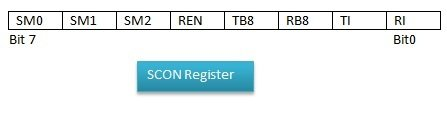SM0:               Serial port mode
Bit 0 of serial port mode.

SM1:               Serial port mode
Bit 1 of serial port mode.

SM2:               Enable multiprocessor
Enable multiprocessor communication in modes 2 and 3 (for 9 bit UART).

Set/clear by software to enable/disable receive operation

TB8:               Transmit bit 8
Set or clear by software. The 9 bits will be transmitted in mode 2 and 3.
TB8 = 1, a value is written to the serial port, 9th bit of data = 1.
TB8 = 0, 9th bit of data = 0, RI will not set.

Set or clear by software. The 9 bits will be received in mode 2 and 3. First eight bits are the data received and 9th bit received will be placed in RB8.

TI:                   Transmit Interrupt flag
Set by hardware when a byte is transmitted completely. Now the port is free and ready to send the next byte. This bit must be cleared by software.

Set by hardware when a byte has been completely received. This lets the program to know that it needs to read the value quickly before another byte is read. This bit must be cleared by software.

### CONFIGURATION SETTINGS

• Setting Operation Mode of Serial Port:

The Upper four bits are configuration bits. SM0 and SM1 set the serial mode between 0 and 3. The four modes along with the baud rates are in the table given below. In modes 0 and 2 the baud rate is fixed which is based on the oscillator frequency. In modes 1 and 3 the baud rate is variable which depends on how often Timer 1 overflows.

SM0 SM1 MODE Description Baud rate

0 0 0 shift register (Fosc./12)
0 1 1 8 bit UART Variable (set by Timer 1)
1 0 2 9 bit UART (Fosc./64)
1 1 3 9 bit UART Variable (set by Timer 1)

SM2 is a flag bit for Multiprocessor communication. When SM2 is set, the 8051 microcontroller will set the RI (Receive Interrupt) flag whenever a byte is received (if the 9th bit received is “1”).  By this way, program knows that a byte has been received and it needs to be processed. If 9th bit is clear, the RI flag will never be set. We will clear this RI bit so that the flag is set upon reception of any character.

If SM2=1, RI=1 (only if 9th bit of received byte is 1)

REN is Receiver Enable bit. Set this bit if we want to receive data through serial port.

REN=1 (for serial communication)

• Setting the Baud Rate of Serial Port:

Baud Rate:

It is defined as number of bits transmitted or received per second and is usually expressed in Bits per second bps. After setting the operation mode of serial port, the program must configure the serial ports baud rate which is only applicable to Serial Port modes 1 and 3. For mode 0 and 2 oscillator frequency determines the Baud Rate.

For mode 0:

Baud rate is always the oscillator frequency divided by 12. For crystal of frequency 11.059MHz, Baud rate will be 921,583 baud.

Baud rate = (11.0592MHz/12) = 921,583 baud

For mode 2:

Baud rate is always the oscillator frequency divided by 64. For crystal of frequency 11.059MHz, Baud rate will be 172,797 baud.

Baud rate ==  (11.0592MHz/64)  = 172,797 baud

For mode 1 and 3:

For them, the baud rate is determined by how frequently timer 1 overflows. The more frequent timer 1 overflows, the higher the baud rate. There are many ways due to which timer 1 overflows at a rate that determines a baud rate. The most common method is:

• We put timer 1 in 8-bit auto-reload mode (timer mode 2).
• This 8 bit timer that allows only values of 00 to FFH loaded in timer register TH1.
• Set a reload value in TH1 that causes Timer 1 to overflow at a frequency appropriate to generate a baud rate.
• If PCON.7 is clear (SMOD=0), then to generate a given baud rate, the value to be put in TH1 can be determined from an equation:

TH1 = 256 – ((Crystal / 384) / Baud)

• If the value to be load in TH1 by above equation is not in whole number then we can’t get accurate baud rate. For that case, set PCON.7 (SMOD=1). The baud rate gets double and the equation becomes:

TH1 = 256 – ((Crystal / 192) / Baud)

## How to calculate baud rate for serial communication in 8051 microcontroller

Let us take an example. If we have crystal of frequency 11.059MHz and we want to configure the serial port to 19,200 baud rate, firstly we try equation 1. By using equation 1:

TH1 = 256 – ((Crystal / 384) / Baud)
TH1 = 256 – ((11.059MHz/ 384) / 19200 )
TH1 = 256 – 1.5 = 254.5

By setting 254 and 255, we don’t achieve accurate baud rate so we need to set PCON.7 (SMOD=1). This doubles the baud rate and we will use equation 2 for determining TH1 value. By using equation 2:

TH1 = 256 – ((Crystal / 192) / Baud)
TH1 = 256 – ((11.059MHz/ 192) / 19200)
TH1 = 256 – 3 = 253

Thus by setting TH1 to 253 we get correct frequency for 19,200 baud rate.

## Example of serial communication programming using Mikro C for 8051

If you do not want to do complicated programming using serial communications registers, you can simply use Mikro c compiler which has built in libraries for the UART communication. Now I will explain about UART programming through mikro c and after that I will provide an example of UART in keil compiler.  We can use built-in UART library for handling 8051 serial communication, there are several functions. In this library.

• UART_init() is used for initialization of 8051 UART module
• UART_init() is used for initialization of 8051 UART module
• UART_Write() writes one byte to serial port. UART_Write() writes one byte to serial port
• while UART_Write_Text() can write multiple bytes.
• UART_Set_Active() function is only used in micro-controllers that support more than one UART ports.
```sbit LCD_RS at P2_0_bit;
sbit LCD_EN at P2_1_bit;
sbit LCD_D7 at P2_5_bit;
sbit LCD_D6 at P2_4_bit;
sbit LCD_D5 at P2_3_bit;
sbit LCD_D4 at P2_2_bit;

void main() {
unsigned int sum;
char txt;
P0=0xFF;
P1=0xFF;

uart1_init(9600);
PCON.SMOD=0;
Lcd_init();
lcd_cmd(_LCD_CURSOR_OFF);
lcd_out(1,1,"****************");
uart1_write_text("Uart OK");
while(1){
sum=P0+P1;
wordToStr(sum,txt);
lcd_out(2,1,"P0+P1=");
lcd_out(2,7,txt);
uart1_write_text("P0+P1=");
uart1_write_text(txt);
uart1_write_text("\r\n");
delay_ms(500);
}
}```

In above we are transmitting data through UART port and also displaying same data on LCD. If you don’t know how to display data on LCD, you can check tutorial here. Now lets see the explanation of code.

• First we initialized serial port at 9600 baud rate using UART_Init() function.
• Next we need to Clear SMOD bit of PCON register
• Actually there is some bug in uart_init() function of UART library, it writes HIGH to SMOD bit that causes erroneous baud rate.
• we can send some string to serial port with uart_write_text() function.
• Now in while(1) loop, we are sending “P0+P1” string to serial port with uart_write_text function.
• now we can send same text array to serial port that is being display on character LCD.
• At the end, we are sending new line character, OK code is now ready we can compile and test it.
• It is recommended to select 11.0592MHz crystal frequency for using serial port 11.0592MHz crystal frequency can give exact clock rates for most of the common baud rates for the UART.
• we can use virtual terminal in Proteus for testing serial communication.
• TX pin of 8051 will be connected to RX of virtual terminal.
• In virtual terminal of proteus, you have to enter same baud rate as configured in your code.
• When you run the code, You will see, sum of P0 & P1 is appearing on virtual terminal,

### Example of serial communication 8051 microcontroller in Keil

8051 microcontroller is used in the schematic. Crystal frequency is set to 11.059MHz. Baud rate used is 9600. A virtual terminal is used. The data coming from transmitter pin of controller will be displayed on virtual terminal. The send to the microcontroller is also received on receiver pin of controller which is sent through virtual terminal by pressing specific key. The program is such that the baud rate is set to 9600.

For 9600 baud rate:

TH1 = 256 – ((Crystal / 384) / Baud)
TH1 = 256 – ((11.59MHz/ 384) / 9600 )
TH1 = 256 – 3 = 253

The Hex value of 253 is FD which should be loaded to TH1

• Port 3 pin 0 receiver pin is connected to TX of virtual terminal.
• Port 3 pin 1 transmitter pin is connected to RX of virtual terminal.
• Port 1 lower four pins are connected with 4 LEDs.

PROTEUS SCHEMATIC: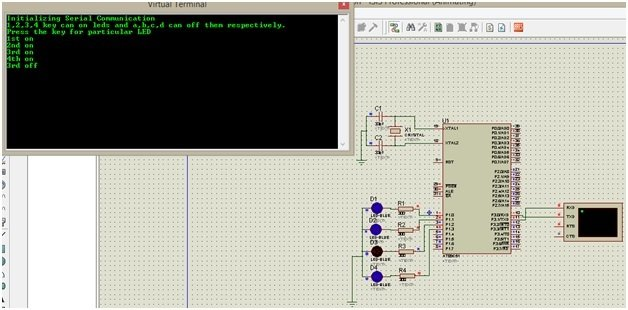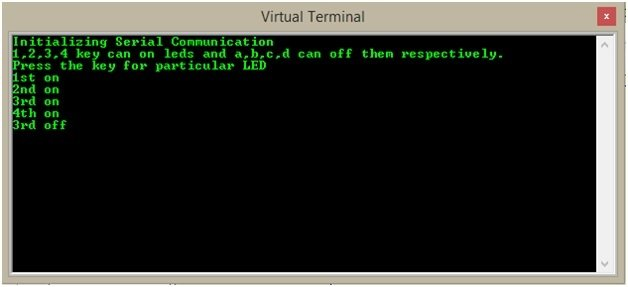### WORKING of serial communication 8051 microcontroller

By pressing key 1, 1st LED will glow and when key “a” is pressed, it goes off.

By pressing key 2, 2nd LED will glow and when key “b” is pressed, it goes off.

By pressing key 3, 3rd LED will glow and when key “c” is pressed, it goes off.

By pressing key 4, 4th LED will glow and when key “d” is pressed, it goes off.

The virtual terminal will also show the ON and OFF of LEDs.

## CODE of serial communication 8051 microcontroller

```#include <REGX51.H>

void cct_init(void);

void SerialInitialize(void);

void uart_msg(unsigned char *c);

void uart_tx(unsigned char);

sbit led1 = P1^0;

sbit led2 = P1^1;

sbit led3 = P1^2;

sbit led4 = P1^3;

void main()

{

cct_init();

SerialInitialize();

EA = 1;

ES = 1;

uart_msg("Initializing Serial Communication");

uart_tx(0x0d);

uart_msg("1,2,3,4 key can on leds and a,b,c,d can off them respectively.");

uart_tx(0x0d);                                                   //next line

uart_msg("Press the key for particular LED");

uart_tx(0x0d);

while(1);

}

void cct_init(void)            //initialize cct

{

P0 = 0x00;                                        //not used

P1 = 0x00;                                        //output port used for leds

P2 = 0x00;                                        //not used

P3 = 0x03;                                        //used for serial communication

}

void SerialInitialize(void)       //Initialize Serial Port

{

TMOD = 0x20;                   //Timer 1 In Mode 2 -Auto Reload to Generate Baud Rate

SCON = 0x50;                    //Serial Mode 1, 8-Data Bit, REN Enabled

TH1 = 0xFD;                       //Load Baud Rate 9600 To Timer Register

TR1 = 1;                              //Start Timer

}

void uart_msg(unsigned char *c)

{

while(*c != 0)

{

uart_tx(*c++);

}

}

void uart_tx(unsigned char serialdata)

{

SBUF = serialdata;                        //Load Data to Serial Buffer Register

while(TI == 0);                               //Wait Until Transmission To Complete

TI = 0;                                                //Clear Transmission Interrupt Flag

}

void serial_ISR (void) interrupt 4

{

if(RI==1)

{

chr = SBUF;

RI = 0;

}

P0 = ~P0;                                         //Show the data has been updated

switch(chr)

{

case '1':  led1 = 1; uart_msg("1st on"); uart_tx(0x0d); break;

case '2':  led2 = 1; uart_msg("2nd on"); uart_tx(0x0d); break;

case '3':  led3 = 1; uart_msg("3rd on"); uart_tx(0x0d); break;

case '4':  led4 = 1; uart_msg("4th on"); uart_tx(0x0d); break;

case 'a':  led1 = 0; uart_msg("1st off"); uart_tx(0x0d);  break;

case 'b':  led2 = 0; uart_msg("2nd off"); uart_tx(0x0d); break;

case 'c':  led3 = 0; uart_msg("3rd off"); uart_tx(0x0d); break;

case 'd':  led4 = 0; uart_msg("4th off"); uart_tx(0x0d); break;

default: ;    break;                        //do nothing

}
RI = 0;

}

```

### 7 thoughts on “serial communication 8051 microcontroller using keil”

1. bldc motor controller with 8051